# SAT 1000 problems series - Upcoming frequently

Now I think it's time to publish all of the 1000 problems of my problem set. They come from some particularly hard, popular or interesting Gaokao problems.

From then on, I will post all of the problems here, the order of the problem would be initially disarranged, but eventually I would complete all of my the problem set.

These problems will follow the same format so that it will be easier for the programs to index them and use them.

I'm thinking using the problem set for my games or other purposes.

Free to use the problem set as a dataset for machine learning or using the problems in your contest, games or programs, just indicate the source :)

Category:

P1-P159 - Functions

P160-P267 - Derivatives

P268-P370 - Trigonometry

P371-P436 - Vectors

P437-P503 - Sequences

P504-P562 - Inequalities

P563-P669 - Solid Geometry

P670-P846 - Analytic Geometry

P847-P861 - Modeling

P862-P941 - Math Insight

P942-P1000 - Miscellaneous

What do you have in Gaokao?

• A pen/pencil

• Draft paper

• Your brilliant mind

What can't you use in Gaokao?

• Calculator (Except for the submit process)

• Numerical method

• Mathematica, Geogebra or other related software/websites

Your pen/pencil, draft paper are the only things you can use. There you go!Note by Alice Smith
1 year ago

This discussion board is a place to discuss our Daily Challenges and the math and science related to those challenges. Explanations are more than just a solution — they should explain the steps and thinking strategies that you used to obtain the solution. Comments should further the discussion of math and science.

When posting on Brilliant:

• Use the emojis to react to an explanation, whether you're congratulating a job well done , or just really confused .
• Ask specific questions about the challenge or the steps in somebody's explanation. Well-posed questions can add a lot to the discussion, but posting "I don't understand!" doesn't help anyone.
• Try to contribute something new to the discussion, whether it is an extension, generalization or other idea related to the challenge.
• Stay on topic — we're all here to learn more about math and science, not to hear about your favorite get-rich-quick scheme or current world events.

MarkdownAppears as
*italics* or _italics_ italics
**bold** or __bold__ bold
- bulleted- list
• bulleted
• list
1. numbered2. list
1. numbered
2. list
Note: you must add a full line of space before and after lists for them to show up correctly
paragraph 1paragraph 2

paragraph 1

paragraph 2

[example link](https://brilliant.org)example link
> This is a quote
This is a quote
    # I indented these lines
# 4 spaces, and now they show
# up as a code block.

print "hello world"
# I indented these lines
# 4 spaces, and now they show
# up as a code block.

print "hello world"
MathAppears as
Remember to wrap math in $$ ... $$ or $ ... $ to ensure proper formatting.
2 \times 3 $2 \times 3$
2^{34} $2^{34}$
a_{i-1} $a_{i-1}$
\frac{2}{3} $\frac{2}{3}$
\sqrt{2} $\sqrt{2}$
\sum_{i=1}^3 $\sum_{i=1}^3$
\sin \theta $\sin \theta$
\boxed{123} $\boxed{123}$

## Comments

Sort by:

Top Newest

If $f(x)$ and $g(x)$ are functions defined at $\mathbb R$, and equation $x-f(g(x))=0$ has real roots.

Then which cannot be the function $g(f(x))$?

$A.\ x^2+x-\dfrac{1}{5}$

$B.\ x^2+x+\dfrac{1}{5}$

$C.\ x^2-\dfrac{1}{5}$

$D.\ x^2+\dfrac{1}{5}$

- 1 year ago

Log in to reply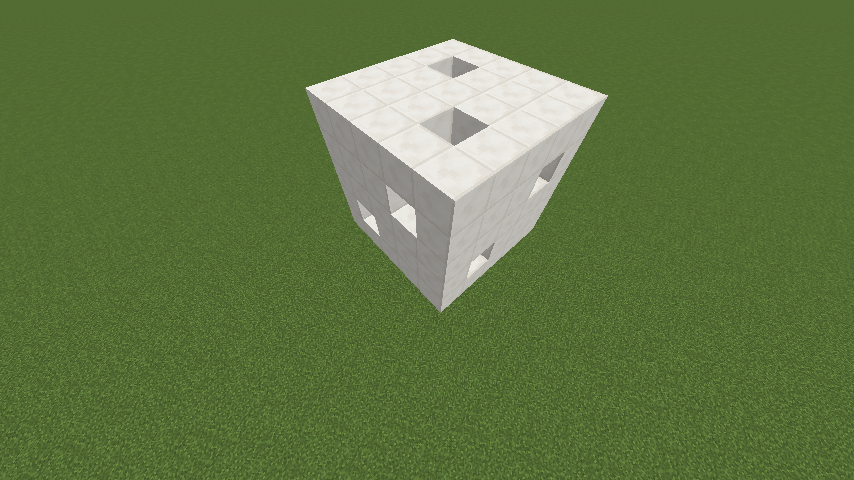As shown above, a $5 \times 5 \times 5$ cube has been punched through so that there will always be two $1 \times 1$ square holes when looking from up, front and left.

Find the surface area of this solid. (Including the interior part)

- 1 year ago

Log in to reply

Total surface area of cube when there is no hole is $6\times 5\times 5 = 150$

On punching $1$ hole, we reduce the surface area of cube's face by $2$(both the ends). So on punching $6$ holes, total of $12$ sq. units area will reduce. So, till now we are left with $150 - 12 = 138$ sq. units of surface area.

Punching holes also generates some surface areas. First consider just one hole. This hole creates $4$ walls each of area $1\times 5 = 5$. So area created by $1$ hole only is $4\times 5 = 20$.

If there were no intersections of holes then calculation was easier. But here there are few intersections of holes. Each hole is intersected by $2$ holes and there are total $6$ holes. So total number of intersections $\displaystyle = \frac{2\cdot 6}{2} = 6$.

Consider a hole $1$ which is intersected by hole $2$ and hole $3$. As said above, hole $1$ will create $20$ sq. units area. But at intersections, hole $2$ and hole $3$ makes additional $2$ holes each in hole $1$ thus reducing the surface area of hole $1$ by $4$. So till now there are $20 - 4 = 16$ sq. units surface in hole $1$.

There are total $6$ holes, so total surface area created $= 16\times 6 = 96$. But at each intersection, $2$ squares of unit square area is counted in both the intersecting holes. That means $2$ sq. units area is counted twice at every intersections. There are $6$ intersections which means total of $12$ sq. units area is counted twice. So we have to subtract this from $96$. So total surface area created by all the six holes is $96 - 12 = 84$.

So total surface area in whole cube will be $138 + 84 = 222$.

- 1 year ago

Log in to reply

There exist function $f(x)$ such that $\forall x \in \mathbb R$, the property follows:

$A.\ f(\sin 2x)=\sin x$

$B.\ f(\sin 2x)=x^2+x$

$C.\ f(x^2+1)=|x+1|$

$D.\ f(x^2+2x)=|x+1|$

- 1 year ago

Log in to reply

Given that $f(x)=\cos 2x, g(x)=\sin x$.

Find all possible real number $a$ and positive integer $n$, so that $f(x)+a g(x)=0$ has exactly $2013$ roots on the interval $(0,n \pi)$.

How to submit:

• First, find the number of all possible solutions $(a,n)$. Let $N$ denote the number of solutions.

• Then sort the solutions by $a$ from smallest to largest, if $a$ is the same, then sort by $n$ from smallest to largest.

• Let the sorted solutions be: $(a_1,n_1), (a_2,n_2), (a_3,n_3), \cdots ,(a_N,n_N)$, then $M=\displaystyle \sum_{k=1}^N k(a_k+n_k)$.

For instance, if the solution is: $(-1,2), (-1,1), (1,3), (0,4)$

Then the sorted solution will be: $(-1,1), (-1,2), (0,4), (1,3)$

Then $N=4$, $M=\displaystyle \sum_{k=1}^4 k(a_k+n_k)= 1 \times (-1+1) + 2 \times (-1+2) + 3 \times (0+4) + 4 \times (1+3) =30$.

For this problem, submit $\lfloor M+N \rfloor$.

- 12 months ago

Log in to reply

If function $f(x)=x-\dfrac{1}{3} \sin 2x + a \sin x$ is monotonic increasing for all $x \in \mathbb R$, what's the range of $a$?

$A.\ [-1,1]$

$B.\ [-1, \dfrac{1}{3}]$

$C.\ [-\dfrac{1}{3}, \dfrac{1}{3}]$

$D.\ [-1, -\dfrac{1}{3}]$

- 11 months ago

Log in to reply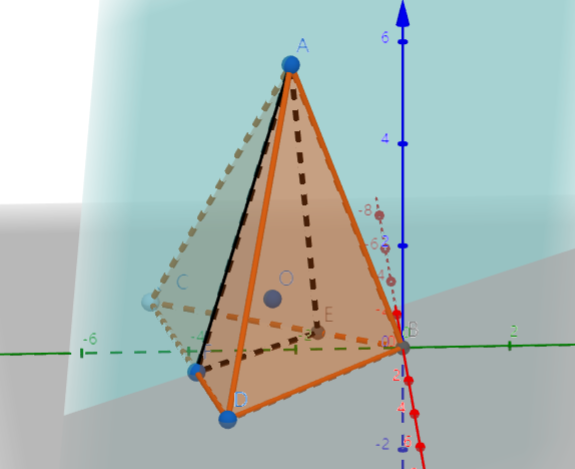As shown above, in triangular pyramid $A-BCD$, the cross section $AEF$ passes through the center of the inscribed sphere $O$ (i.e. The sphere which is tangent to all of the faces of the solid) of $A-BCD$, and it intersects with $BC, DC$ at point $E,F$ respectively.

If the cross section divides the pyramid into two parts whose volumes are equal, $S_1$ is the surface area of solid $A-BEFD$, $S_2$ is the surface area of solid $A-EFC$, what is always true for $S_1$ and $S_2$?

- 1 year ago

Log in to reply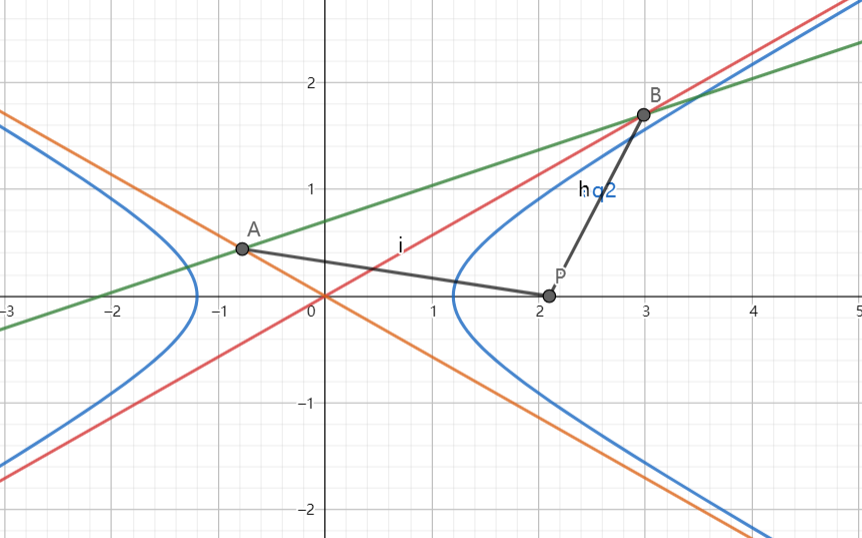As shown above, the line: $x-3y+m=0\ (m \neq 0)$ intersects with the two asymptotes of the hyperbola:

$\dfrac{x^2}{a^2}-\dfrac{y^2}{b^2}=1\ (a>0,b>0)$ at point $A,B$.

If point $P$ is at $(m,0)$ and $|PA|=|PB|$, find the eccentricity of the hyperbola.

Let $E$ denote the eccentricity, submit $\lfloor 1000E \rfloor$.

- 1 year ago

Log in to reply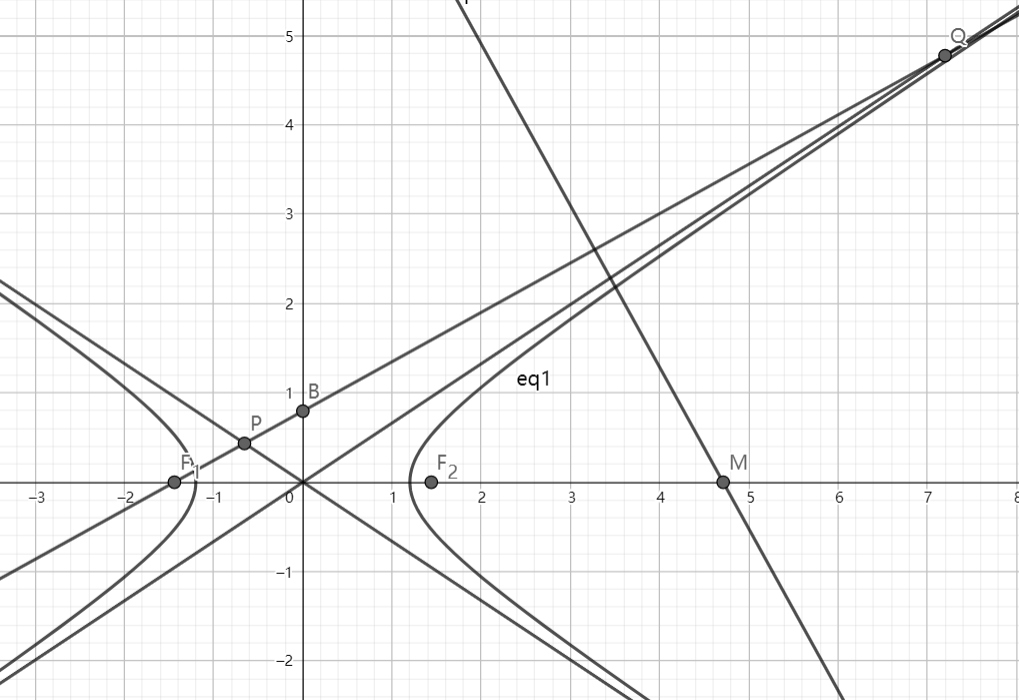As shown above, $F_1, F_2$ are left and right focus of the hyperbola: $\dfrac{x^2}{a^2}-\dfrac{y^2}{b^2}=1\ (a,b>0)$ respectively, and $B(0,b)$.

Line $F_1B$ intersects with the two asymptotes of the hyperbola at $P,Q$, and the perpendicular bisector of $PQ$ intersects with x-axis at point $M$.

If $|MF_2|=|F_1F_2|$, then find the eccentricity of the hyperbola.

Let $E$ denote the eccentricity, submit $\lfloor 1000E \rfloor$.

- 1 year ago

Log in to reply

Let $A,B$ be the end points of the major axis of the ellipse $C:\dfrac{x^2}{3}+\dfrac{y^2}{m}=1$.

If there exists point $M$ on the ellipse so that $\angle AMB = \dfrac{2\pi}{3}$, find the range of $m$.

These pictures show the two cases: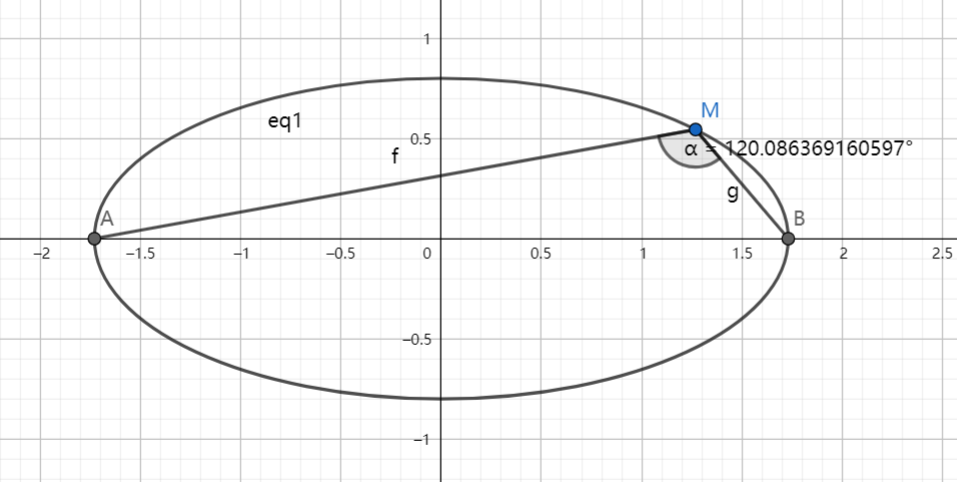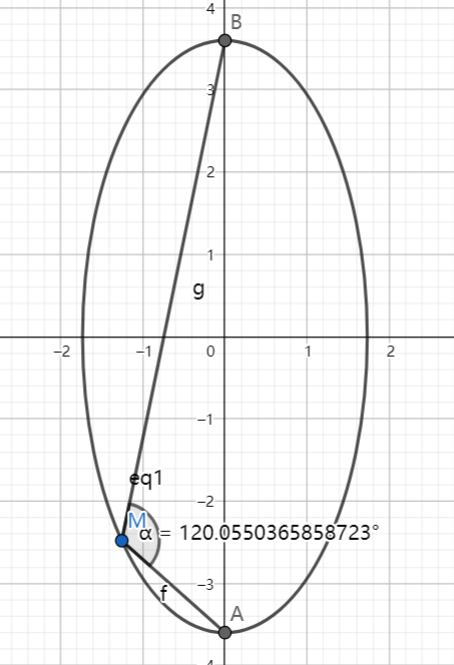- 1 year ago

Log in to reply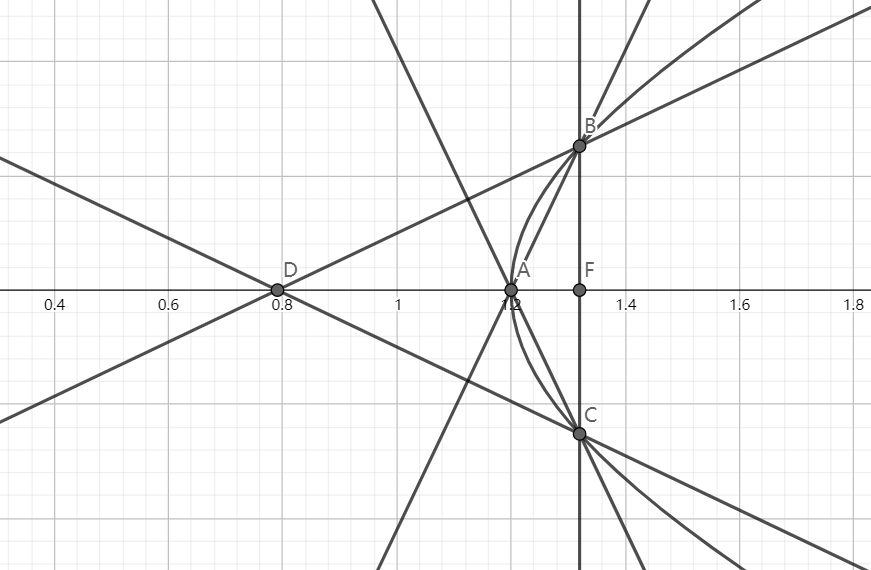As shown above, the hyperbola: $\dfrac{x^2}{a^2}-\dfrac{y^2}{b^2}=1\ (a>0,b>0)$ has right focus point $F$, right vertex $A$.

Line $l_1$ passes through $F$ and it intersects with the hyperbola at point $B,C$, $l_2$ passes through $B$ and $l_2 \perp AC$, $l_3$ passes through $C$ and $l_2 \perp AB$, $l_2, l_3$ intersects at point $D$.

If the distance from $D$ to line $l_1$ is less than $a+\sqrt{a^2+b^2}$, what is the range of the slope of the hyperbola's asymptotes?

- 1 year ago

Log in to reply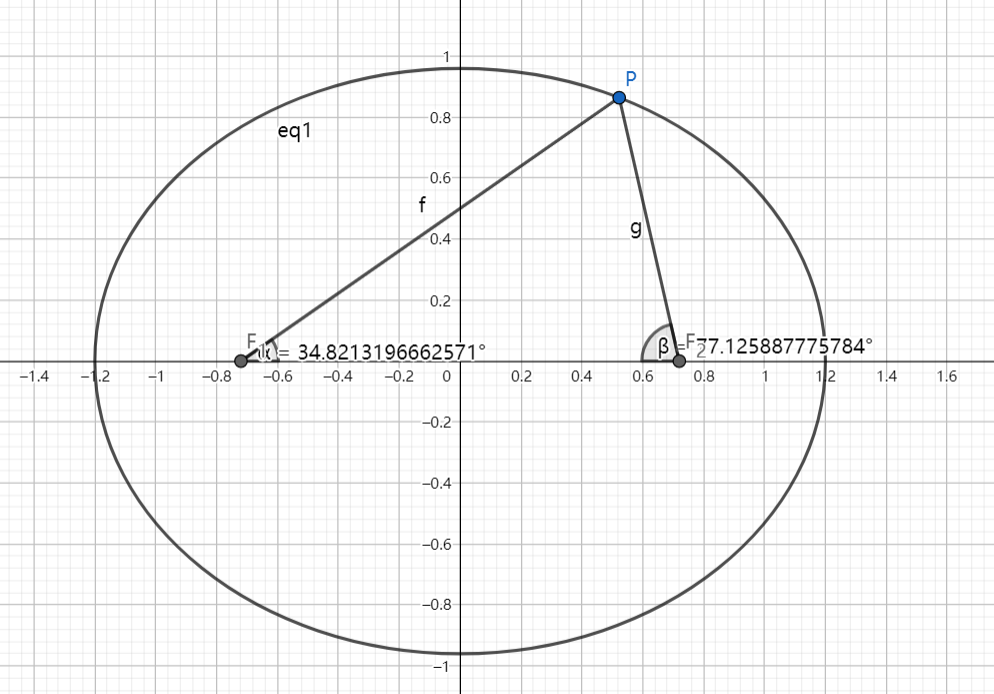As shown above, the left and right focus of the ellipse: $\dfrac{x^2}{a^2}+\dfrac{y^2}{b^2}=1 (a>b>0)$ are $F_1(-c,0), F_2(c,0)$.

If there exists point $P$ such that $\dfrac{a}{\sin \angle PF_1F_2}=\dfrac{c}{\sin \angle PF_2F_1}$, find the range of the eccentricity of the ellipse.

The range can be expressed as $(l,r)$. Submit $\lfloor 1000(2r-l) \rfloor$.

- 1 year ago

Log in to reply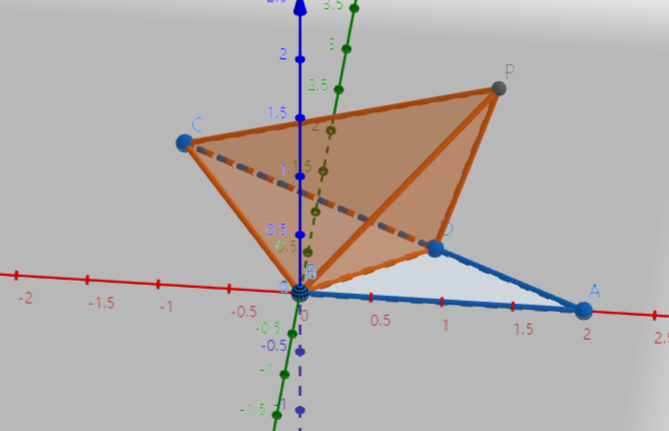As shown above, in $\triangle ABC$, $AB=BC=2$, $\angle ABC = \dfrac{2\pi}{3}$.

If $P$ is outside plane $ABC$ and point $D$ is on segment $AC$, so that $PD=DA, PB=BA$, then find the maximum volume of pyramid $PBCD$.

Let $V$ denote the volume of $PBCD$, submit $\lfloor 10000V \rfloor$.

- 1 year ago

Log in to reply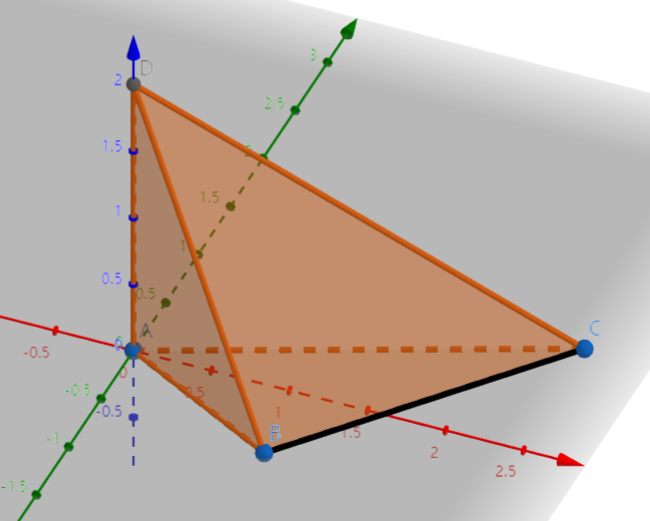As shown above, in pyramid $ABCD$, $AD \perp BC$, if $AD=BC=2$, $AB+BD=AC+CD=4$, then find the maximum volume for pyramid $ABCD$.

Let $V$ denote the maximum volume. Submit $\lfloor 1000V \rfloor$.

- 1 year ago

Log in to reply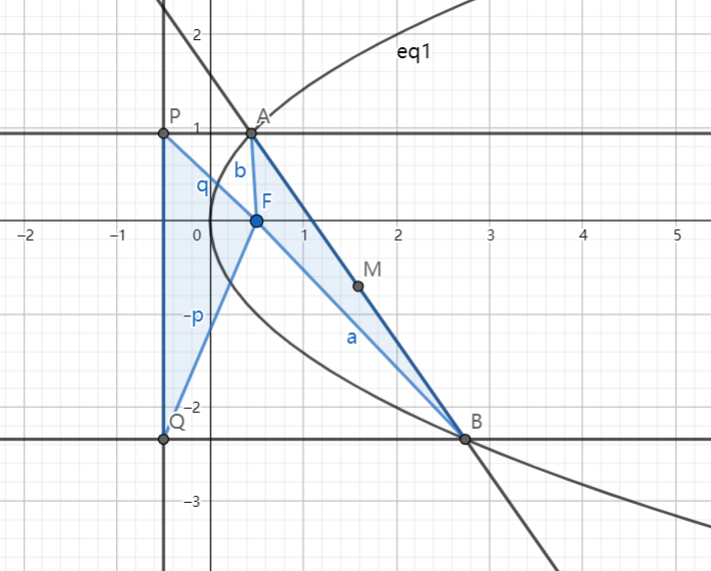As shown above, the focus of the parabola $C: y^2=2x$ is $F$. Two lines $l_1, l_2$ which are parallel to the x-axis intersect with $C$ at $A,B$ and intersect with the directix at $P,Q$. $M$ is the midpoint of $AB$.

If $S_{\triangle PQF}=2S_{\triangle ABF}$, find the locus of point $M$.

If the locus can be expressed as $f(x,y)=0$, then when $y=50$, submit the sum of all possible value(s) for $x$.

Note: $S_{\triangle ABC}$ denotes the area of $\triangle ABC$.

- 1 year ago

Log in to reply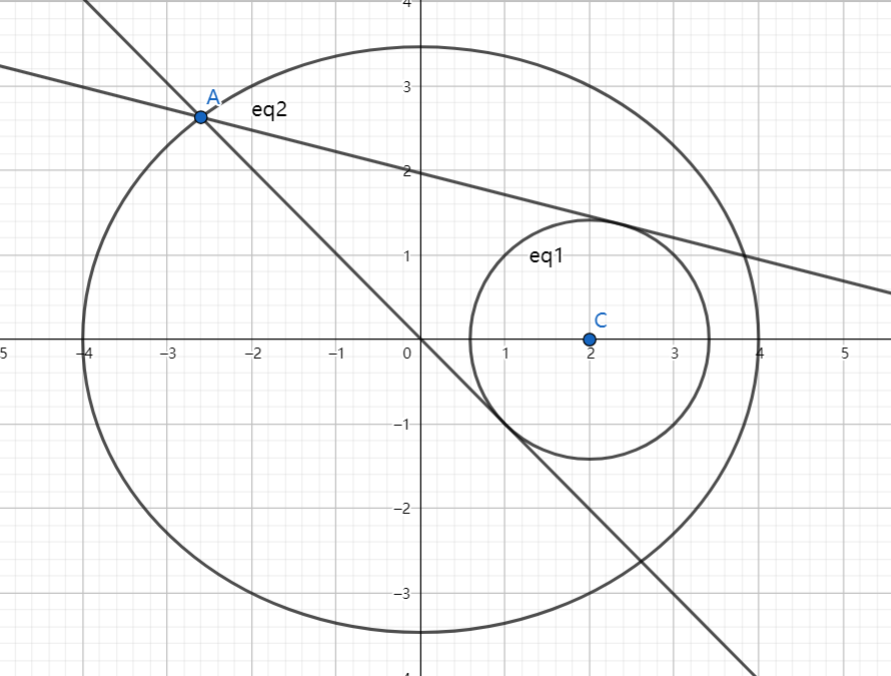As shown above, the ellipse $E$ has equation: $\dfrac{x^2}{16}+\dfrac{y^2}{12}=1$, and point $C$ is the center of the circle: $x^2+y^2-4x+2=0$ If $P$ is a point on ellipse $E$, $l_1, l_2$ both pass through $P$ and they are both tangent to circle $C$, and the product of the slope of $l_1, l_2$ is equal to $\dfrac{1}{2}$, then find the all possible coordinate(s) of point $P$.

How to submit:

• First, find the number of all possible solutions. Let $N$ denote the number of solutions.

• Then Sort the solutions by x-coordinate from smallest to largest, if the x-coordinate is the same, then sort by y-coordinate from smallest to largest.

• Let the sorted solutions be: $(x_1,y_1), (x_2,y_2), (x_3,y_3), \cdots ,(x_N,y_N)$, then $M=\displaystyle \sum_{k=1}^N k(x_k+y_k)$.

For instance, if the solution is: $(-1,2), (-1,1), (1,3), (0,4)$

Then the sorted solution will be: $(-1,1), (-1,2), (0,4), (1,3)$

Then $N=4$, $M=\displaystyle \sum_{k=1}^4 k(x_k+y_k)= 1 \times (-1+1) + 2 \times (-1+2) + 3 \times (0+4) + 4 \times (1+3) =30$.

For this problem, submit $\lfloor 1000(M+N) \rfloor$.

- 1 year ago

Log in to reply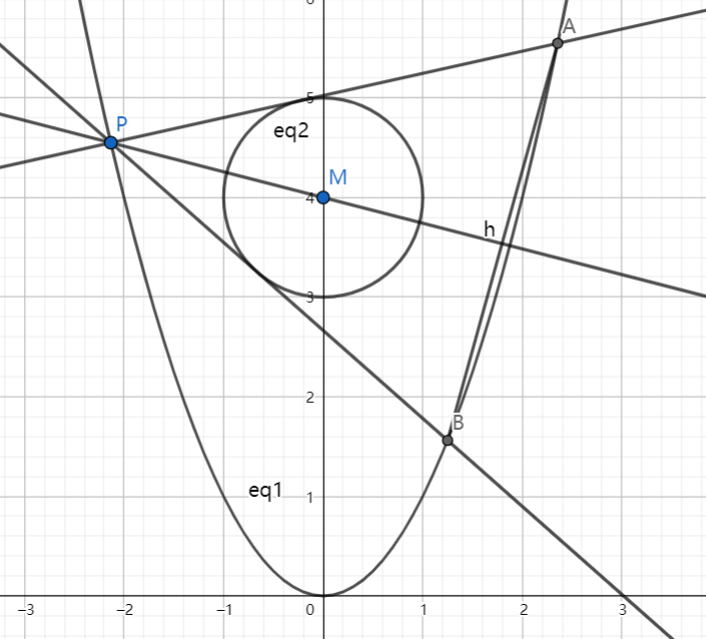As shown above, the parabola $C_1: x^2=y$, circle $C_2: x^2+{(y-4)}^2=1$, and $M$ is the center of circle $C_2$.

Point $P$ is a point on $C_1$ (not at $(0,0)$), and $l_1, l_2$ are two lines tangent to $C_2$ and they intersects with $C_1$ at point $A,B$ respectively. Line $l$ passes through $M$ and $P$.

If $l \perp AB$, find the equation of line $l$.

The equation can be expressed as: $y=\pm k x+b\ (k>0)$. Submit $\lfloor 1000(k+b) \rfloor$.

- 1 year ago

Log in to reply

Can you do some SAT algebra questions? @Alice Smith

Log in to reply

They are on my plan. There you go:)

Of course, they are not really SAT problems, but adapted from GaoKao problems. If you can do them all, you can definitely ace the SAT:)

- 1 year ago

Log in to reply

Let $f_1(x)=x^2, f_2(x)=2(x-x^2), f_3(x)=\dfrac{1}{3}|\sin 2\pi x|$.

If $I_k=\displaystyle \sum_{i=1}^{99} |f_k(\dfrac{i}{99})-f_k(\dfrac{i-1}{99})|$, compare $I_1, I_2, I_3$.

$A.\ I_1

$B.\ I_2

$C.\ I_1

$D.\ I_3

- 1 year ago

Log in to reply

Given that in $\triangle ABC$, $\sin 2A+\sin(A-B+C)=\sin(C-A-B)+\dfrac{1}{2}$, $S$ is the area of $\triangle ABC$, $1 \leq S \leq 2$, let $a,b,c$ be the opposite side of angle $A,B,C$ respectively.

Which inequality always holds?

$A.\ bc(b+c)>8$

$B.\ ab(a+b)>16 \sqrt{2}$

$C.\ 6 \leq abc \leq 12$

$D.\ 12 \leq abc \leq 24$

- 1 year ago

Log in to reply

Given that $a,b \in \mathbb R, a+b=2, b>0$, then find the minimum value of $\dfrac{1}{2|a|}+\dfrac{|a|}{b}$.

Let $M$ be the minimum value. Submit $\lfloor 1000M \rfloor$.

- 1 year ago

Log in to reply

Given that $x,y \in \mathbb R$ and $x^2+y^2 \leq 1$, find the minimum value of $|2x+y-2|+|6-x-3y|$.

Let $M$ be the minimum value. Submit $\lfloor 1000M \rfloor$.

- 1 year ago

Log in to reply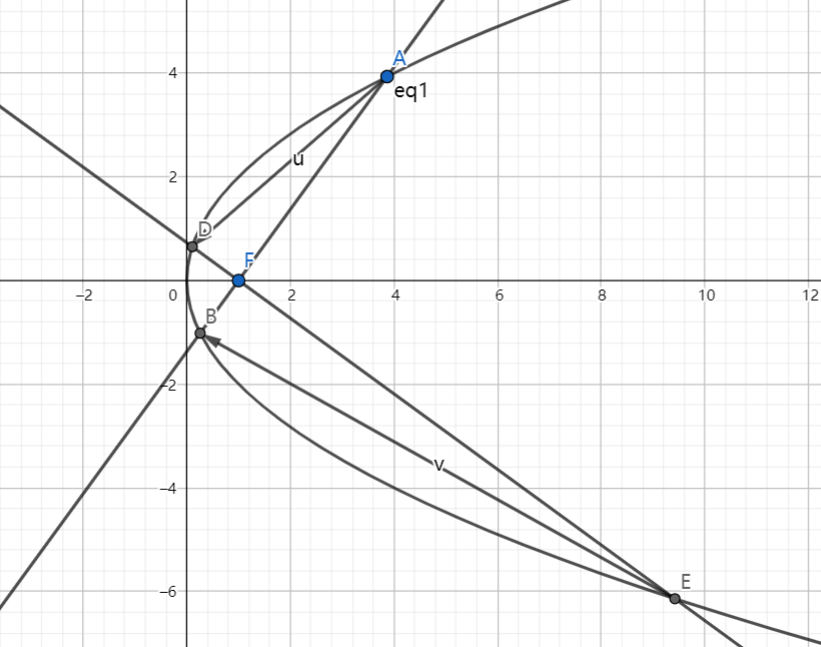As shown above, parabola $C$ has equation: $y^2=4x$ and its focus is $F$.

Line $l_1, l_2$ both passes through $F$, $l_1$ intersects with $C$ at $A,B$, $l_2$ intersects with $C$ at $D,E$, $l_1 \perp l_2$.

Then find the minimum value of $\overrightarrow{AD} \cdot \overrightarrow{EB}$.

Let $M$ be the minimum value. Submit $\lfloor 1000M \rfloor$.

- 1 year ago

Log in to reply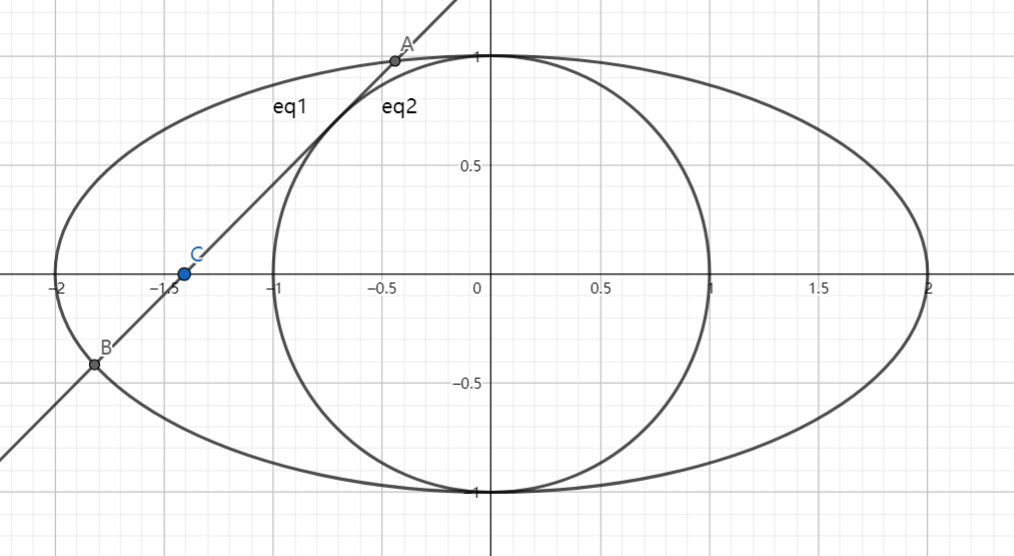As shown above, the ellipse has equation: $\dfrac{x^2}{4}+y^2=1$.

If line $l$ passes through point $C(m,0)$ and is tangent to circle: $x^2+y^2=1$.

$l$ intersects with the ellipse at point $A,B$, then find the maximum value of $|AB|$.

Let $M$ be the maximum value. Submit $\lfloor 1000M \rfloor$.

- 1 year ago

Log in to reply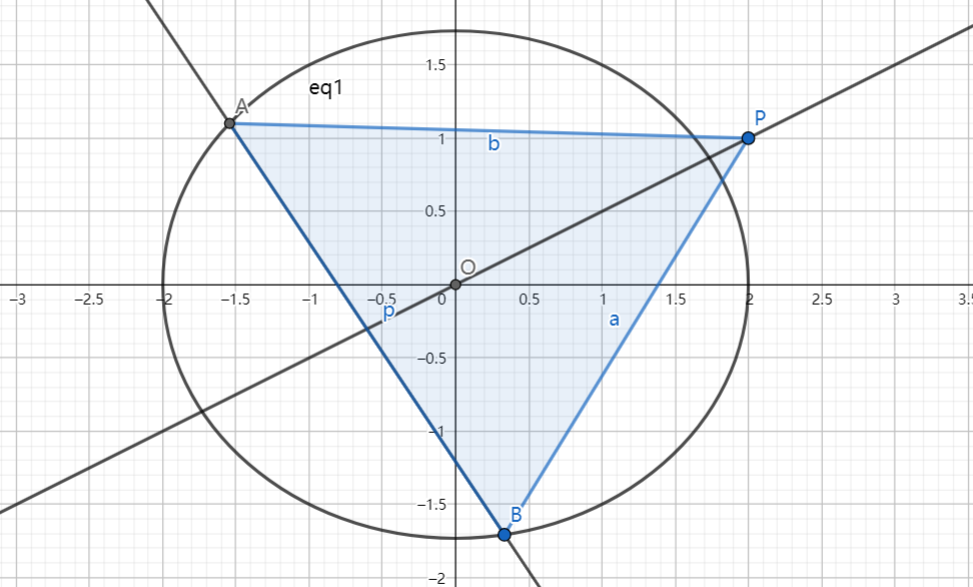As shown above, the ellipse $C: \dfrac{x^2}{4}+\dfrac{y^2}{3}=1$, $O(0,0), P(2,1)$, line $l$ intersects with $C$ at point $A,B$, and line $OP$ bisects segment $AB$.

If the area of $\triangle APB$ reaches the maximum value, then find the equation of line $l$.

The equation of the line can be expressed as $y=kx+b$, submit $\lfloor 1000(k+b) \rfloor$.

- 1 year ago

Log in to reply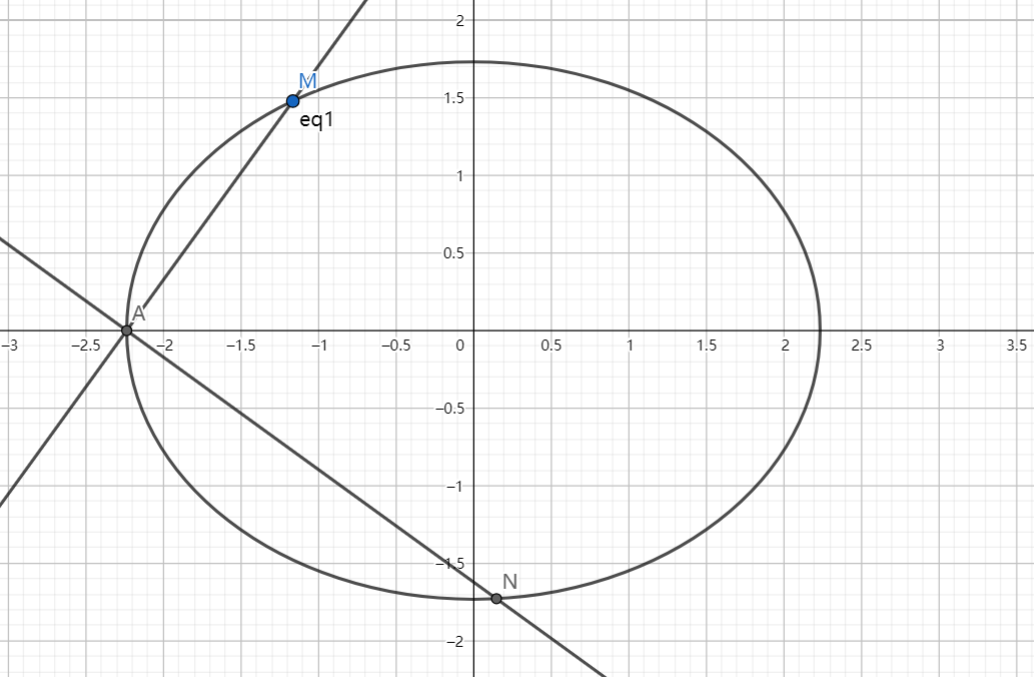As shown above, given that ellipse $E: \dfrac{x^2}{t}+\dfrac{y^2}{3}=1\ (t>3)$, point $A$ is the left vertex of $E$, and line $l$ whose slope is $k\ (k>0)$ intersects with $E$ at point $A,M$, point $N$ is a point on $E$ such that $MA \perp NA$.

If $2|AM|=|AN|$, find the range of $k$ as $t$ changes.

If the range can be expressed as $(l,r)$, submit $\lfloor 1000(2r-l) \rfloor$.

- 1 year ago

Log in to reply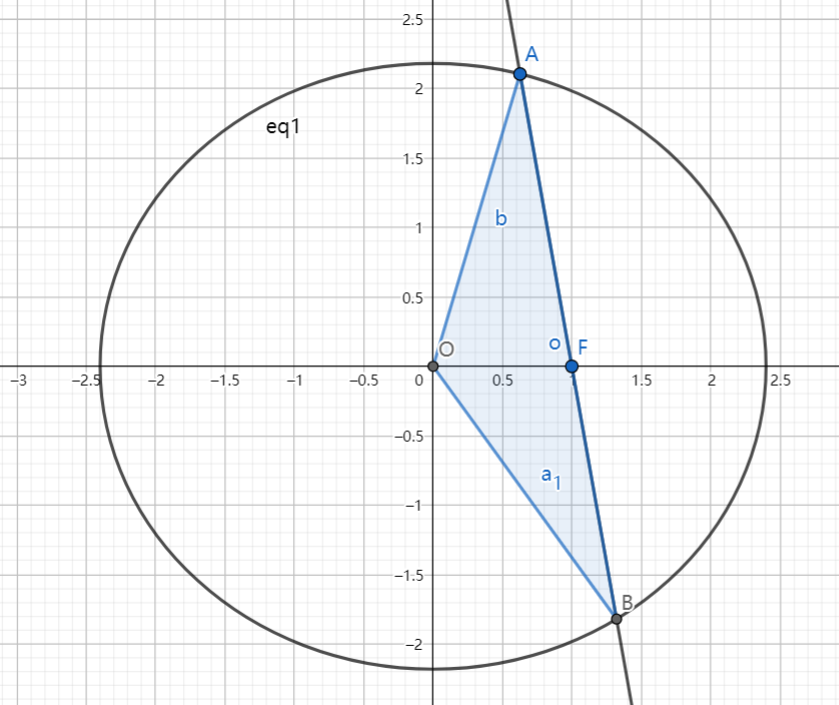As shown above, given that $F(1,0)$ is the right focus of the ellipse: $\dfrac{x^2}{a^2}+\dfrac{y^2}{b^2}=1\ (a>b>0)$, $O$ is the origin.

If for all lines passing through $F$ which intersect with the ellipse at point $A,B$, the following inequality always holds:

$|OA|^2+|OB|^2<|AB|^2$

Then find the range of $a$.

If the range can be expressed as: $(l,+\infty)$, submit $\lfloor 1000l \rfloor$.

- 1 year ago

Log in to reply

Find the range of the function $f(x)=\dfrac{\sin x-1}{\sqrt{3-2\cos x-2\sin x}}\ (x \in [0,2\pi])$.

If the range can be expressed as $[l,r]$, submit $\lfloor 1000(2r-l) \rfloor$.

- 1 year ago

Log in to reply

Note: It is identical to Let's get a little trigy, since it happens to be on my problem set, I decided to repost it.

- 1 year ago

Log in to reply

Given the function $f(x)= \sin(\omega x+\phi)\ (\omega>0, |\phi| \leq \dfrac{\pi}{2})$, $f(-\dfrac{\pi}{4})=0, f'(\dfrac{\pi}{4})=0$, and $f(x)$ is strictly monotone on the interval $(\dfrac{\pi}{18}, \dfrac{5\pi}{36})$, then find the maximum value of $\omega$.

- 1 year ago

Log in to reply

Given the function $f(x)=\sin(\omega x + \dfrac{\pi}{3})\ (\omega>0)$, $f(\dfrac{\pi}{6})=f(\dfrac{\pi}{3})$.

If $f(x)$ has minimum value but no maximum value on the interval $(\dfrac{\pi}{6},\dfrac{\pi}{3})$, then find the value of $\omega$.

If $\omega=\dfrac{a}{b}$, where $a,b$ are positive coprime integers. submit $a+b$.

- 1 year ago

Log in to reply

Given the function $f(x)=\sin^2 \dfrac{\omega x}{2}+\dfrac{1}{2} \sin \omega x - \dfrac{1}{2}\ (\omega>0)$, $x \in \mathbb R$.

If $f(x)=0$ has no roots for $x \in (\pi, 2\pi)$, then find the range of $\omega$.

$A.\ (0,\dfrac{1}{8}]$

$B.\ (0,\dfrac{1}{4}] \cup [\dfrac{5}{8},1]$

$C.\ (0,\dfrac{5}{8}]$

$D.\ (0,\dfrac{1}{8}] \cup [\dfrac{1}{4},\dfrac{5}{8}]$

- 1 year ago

Log in to reply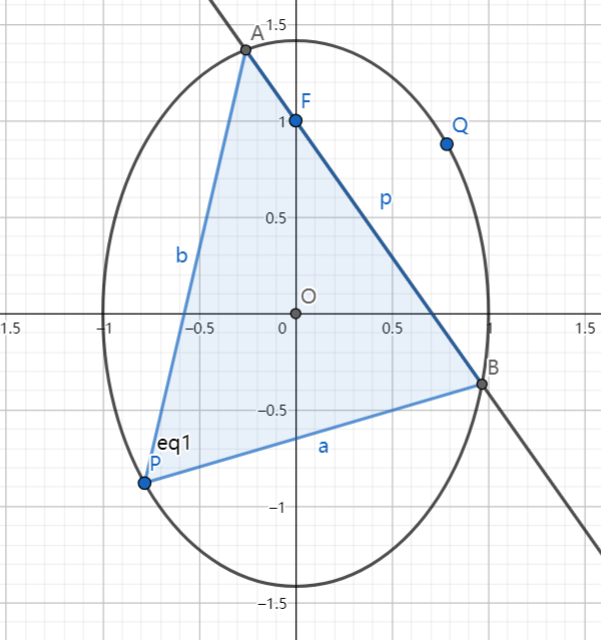As shown above, the ellipse has equation: $x^2+\dfrac{y^2}{2}=1$, $F(0,1)$, line $l: y=-\sqrt{2} x + 1$ intersects with the ellipse at point $A,B$, and $P$ is a point on the ellipse so that $\overrightarrow{OA}+\overrightarrow{OB}+\overrightarrow{OP}=\textbf 0$.

Point $P,Q$ are symmetry about point $O$, and it turns out $A,P,B,Q$ are on the same circle.

The radius of the circle is $r$. Submit $\lfloor 1000r \rfloor$.

- 1 year ago

Log in to reply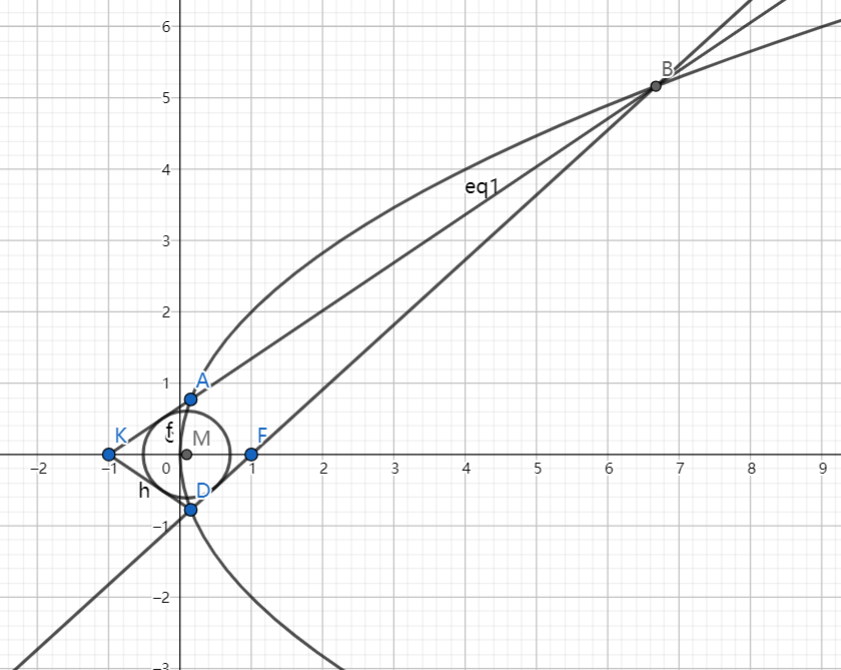As shown above, point $F$ is the focus of the parabola $C: y^2=4x$, and line $l$ passing through $K(-1,0)$ intersects with $C$ at point $A,B$, and $A,D$ are symmetry about the x-axis.

If $\overrightarrow{FA} \cdot \overrightarrow{FB}=\dfrac{8}{9}$, then find the equation of the incircle of $\triangle BDK$.

The equation can be expressed as: $(x-a)^2+(y-b)^2=r^2$. Submit $\lfloor 1000(a+b+r) \rfloor$.

- 1 year ago

Log in to reply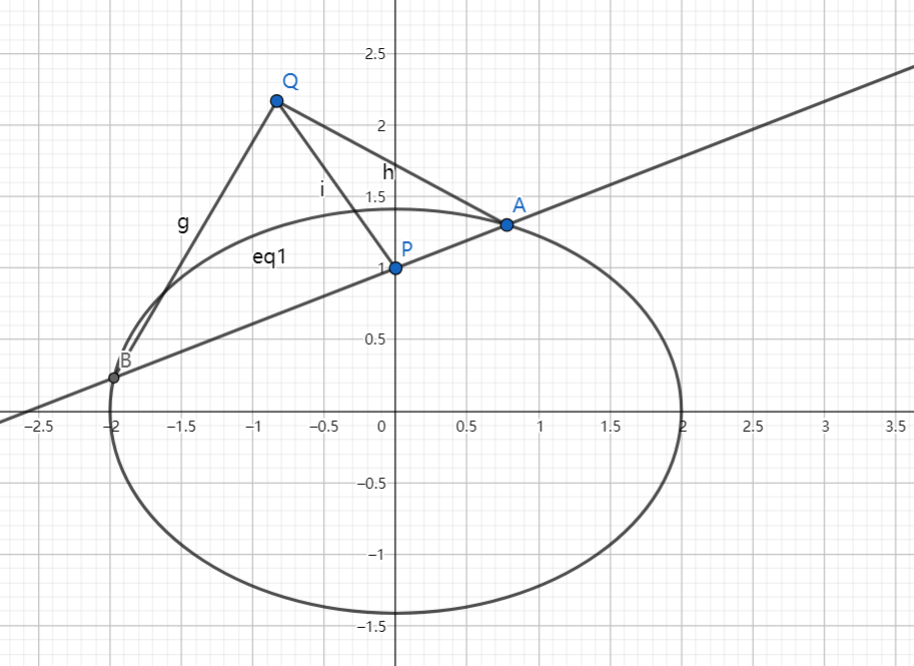As shown above, the ellipse has equation: $\dfrac{x^2}{4}+\dfrac{y^2}{2}=1$, and line $l$ passing through $P(0,1)$ intersects with the ellipse at point $A,B$.

Then there exists a fixed point $Q$ so that the following equation always holds as $l$ rotates:

$\dfrac{|QA|}{|QB|}=\dfrac{|PA|}{|PB|}$

Then find the coordinate of $Q$.

The coordinate of $Q$ is $(x_0,y_0)$. Submit $\lfloor 1000(2y_0-x_0) \rfloor$.

- 1 year ago

Log in to reply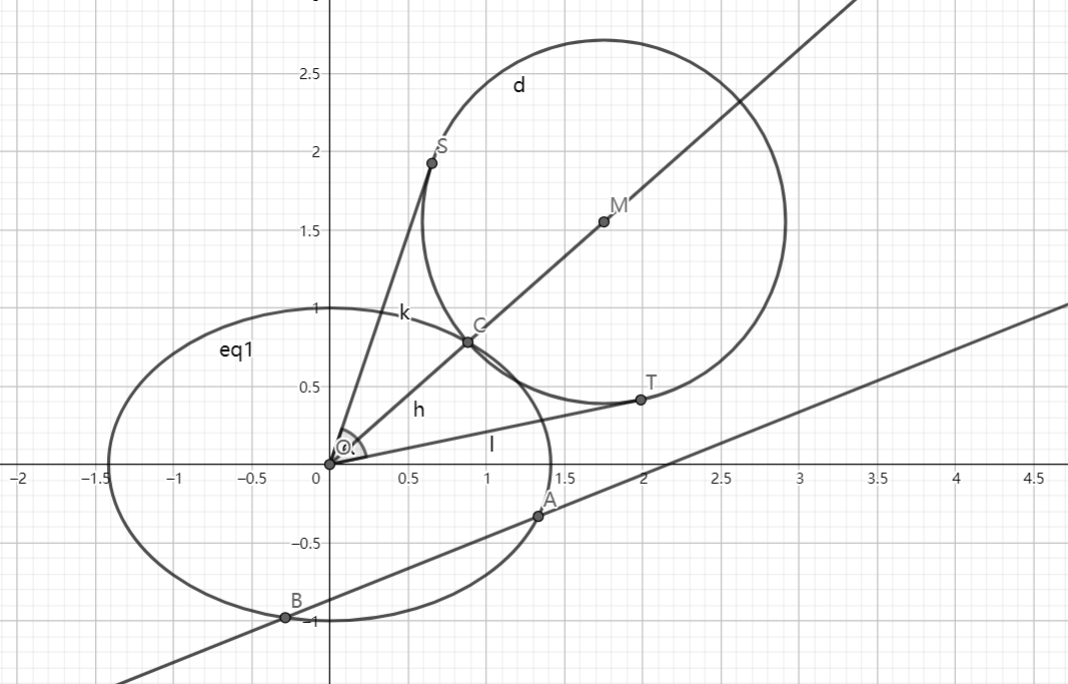As shown above, the ellipse has equation: $\dfrac{x^2}{2}+y^2=1$, line $l: y=k_1 x-\dfrac{\sqrt{3}}{2}$ intersects with the ellipse at point $A,B$.

Point $C$ is on the ellipse and line $OC$ has slope $k_2$, $k_1 k_2=\dfrac{\sqrt{2}}{4}$.

$M$ is a point on ray $OC$, $|MC| : |AB| = 2 : 3$, and the radius of circle $M$ is $|MC|$, $OS,OT$ are two tangent lines of circle $M$ and $S,T$ are tangent points.

Then find the maximum value of $\angle SOT$ (in radians), and find the slope of $l$ when $\angle SOT$ reaches the maximum.

Let $\theta$ be the maximum value of $\angle SOT$ (in radians), $k$ is the slope of $l$. Submit $\lfloor 1000(\theta+|k|)\rfloor$.

- 1 year ago

Log in to reply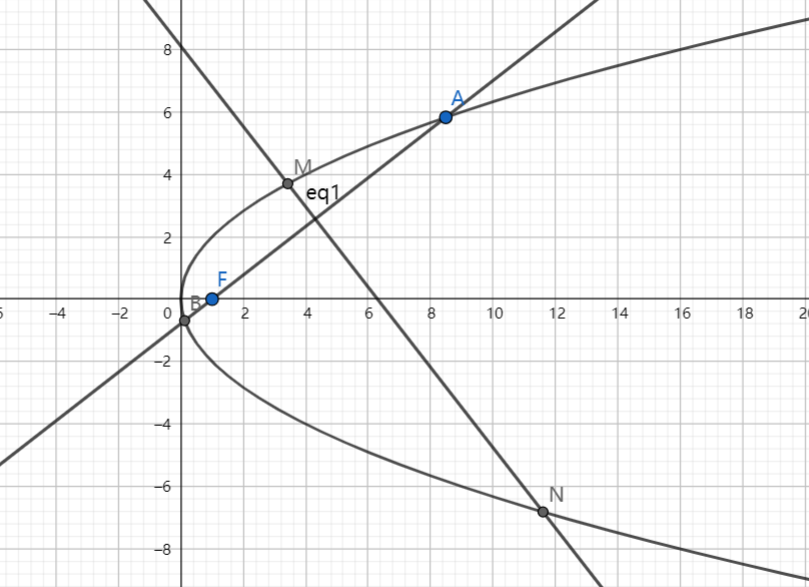As shown above, $F(1,0)$ is the focus of the parabola $C: y^2=4x$, line $l: y=k(x-1)$ intersects with $C$ at point $A,B$, and the perpendicular bisector of $AB$ intersects with $C$ at point $M,N$.

If $A,M,B,N$ are on the same circle, then find the value of $\lfloor 1000|k| \rfloor$.

- 1 year ago

Log in to reply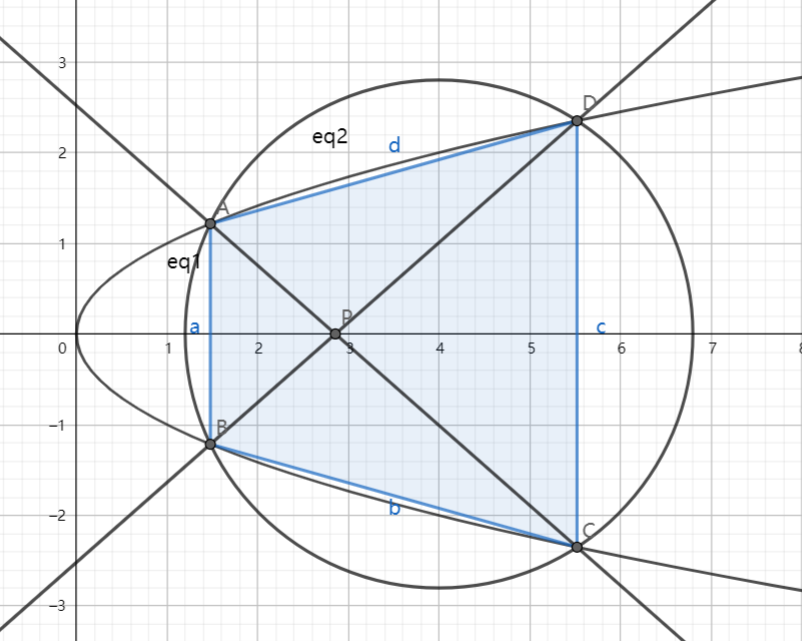As shown above, the parabola $E: y^2=x$ and circle $M: (x-4)^2+y^2=r^2$ for $r>0$ intersect at points $A$, $B$, $C$, and $D$; and their relative positions are shown in the figure. Lines $AC$ and $BD$ intersect at point $P$.

Find the coordinates $(x_0,0)$ of $P$ as the area of quadrilateral $ABCD$ reaches the maximum when $r$ varies. Submit $\lfloor 1000x_0 \rfloor$.

The coordinate of point $P$ is $(x_0,0)$. Submit $\lfloor 1000x_0 \rfloor$.

- 1 year ago

Log in to reply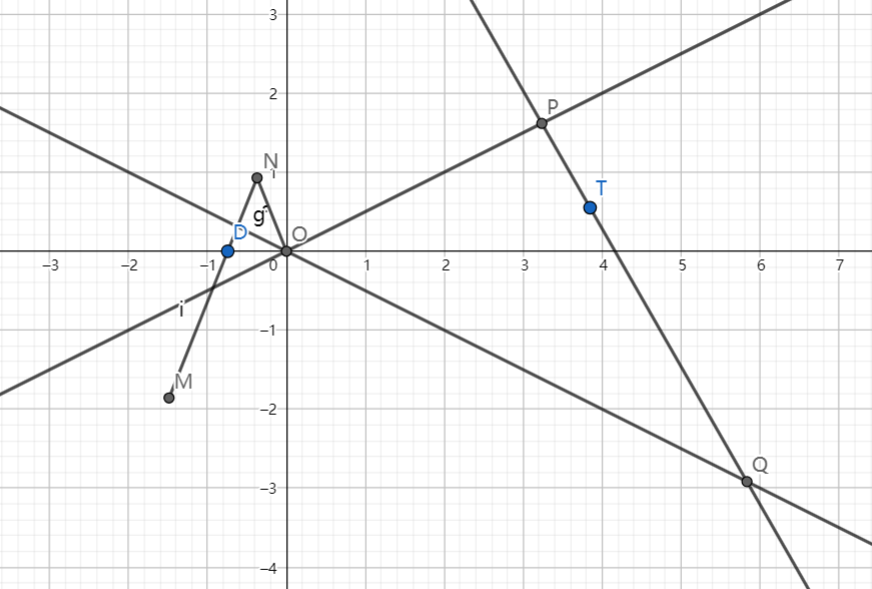As shown above, $ON, NM$ are rigid rods and $DN=ON=1,MN=3$, $O(0,0)$ is fixed on the coordinate plane, and $D$ is restricted along the x-axis. Then as $D$ moves horizontally, point $M$ will rotate around point $O$. Curve $C$ is the locus of point $M$.

If line $l$ intersects with $l_1:x-2y=0$ at point $P$, $l_2:x+2y=0$ at point $Q$, and $l$ is tangent to curve $C$.

Then find the minimum area of $\triangle OPQ$ when line $l$ moves and rotates.

Let $S$ be the minimum area. Submit $\lfloor 1000S \rfloor$.

- 12 months ago

Log in to reply

Given that $f(x)=\dfrac{1}{1+x}$. $\{a_n\}$ is a sequence whose terms are all positive so that $a_1=1, a_{n+2}=f(a_n)$.

If $a_{2010}=a_{2012}$, find the value of $\lfloor 1000(a_{20}+a_{11}) \rfloor$.

- 12 months ago

Log in to reply

$\{a_n\}$ is a sequence such that $a_{n+1}+(-1)^n a_n=2n-1$, then find $\displaystyle \sum_{k=1}^{60} a_k$.

- 12 months ago

Log in to reply

Given that $a_n=n^2(\cos^2 \dfrac{n\pi}{3}-\sin^2 \dfrac{n\pi}{3})\ (n \in \mathbb N^+)$, let $S_n=\displaystyle \sum_{k=1}^{n} a_k$.

$b_n=\dfrac{S_{3n}}{n \cdot 4^n}\ (n \in \mathbb N^+)$, $T_n=\displaystyle \sum_{k=1}^{n} b_k$.

$T_{10} = \dfrac{p}{q}$, where $p,q$ are positive coprime integers.

Submit $\lfloor p-q+2(S_{100}+S_{201}+S_{302}) \rfloor$.

- 12 months ago

Log in to reply

6324366?

- 3 months, 3 weeks ago

Log in to reply

$\{a_n\}$ is a sequence such that $a_1=m\ (m \in \mathbb N^+)$, $a_{n+1}=\begin{cases} \dfrac{a_n}{2} ,\ a_n \equiv 0 \pmod{2} \\ 3 a_n+1 ,\ a_n \equiv 1 \pmod{2} \end{cases}$ If $a_6=1$, find the sum of all possible value(s) for $m$.

- 12 months ago

Log in to reply

Given that $\{a_n\}$ is a Geometric Sequence, its common ratio $q=\sqrt{2}$, $S_n=\displaystyle \sum_{k=1}^n a_k$.

Let $T_n=\dfrac{17 S_n - S_{2n}}{a_{n+1}}\ (n \in \mathbb N^+)$. If $T_{m}$ is the maximum term of sequence $\{T_n\}$, find $m$.

- 12 months ago

Log in to reply

$f(x)$ is a function defined at $[0,1]$ such that:

• $f(0)=f(1)=0$.

• $\forall x,y \in [0,1]\ (x \neq y), |f(x)-f(y)| < \dfrac{1}{2} |x-y|$.

If $\forall x,y \in [0,1], |f(x)-f(y)|, find the minimum value of $k$.

Let $K$ be the minimum value. Submit $\lfloor 1000K \rfloor$.

- 12 months ago

Log in to reply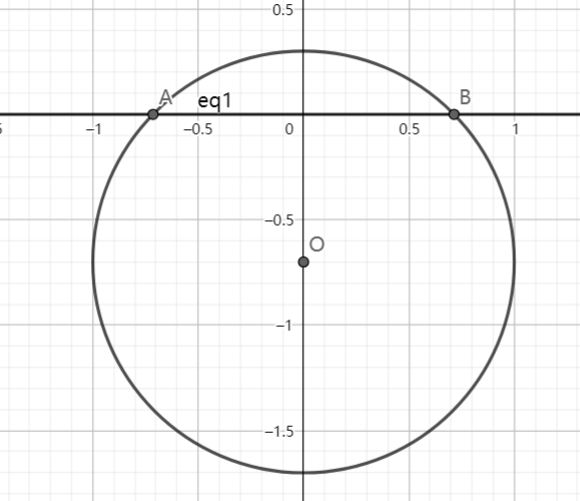As shown above, the circle has radius $r=1\ m$, $O$ is its center. At $t=0$, $O$ is at $(0,-1)$, and it is moving at $v=1\ m/s$ upwards along the y-axis. Let $x(t)$ be the length of the arc above the x-axis, $f(t)=\cos (x(t))$.

For $0 \leq t \leq 1$, what is the best graph for $f(t)$?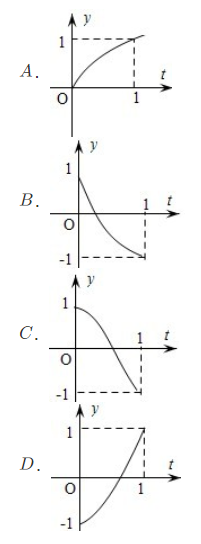- 12 months ago

Log in to reply

Without using calculator, Find $\dfrac{\cos 10 \degree}{\tan 20 \degree}+\sqrt{3} \sin 10 \degree \tan 70 \degree - 2 \cos 40 \degree$.

Let $A$ denote the answer. Submit $\lfloor 1000A \rfloor$.

- 12 months ago

Log in to reply

If $\tan \alpha=2 \tan \dfrac{\pi}{5}$, find $\dfrac{\cos(\alpha-\dfrac{3\pi}{10})}{\sin(\alpha-\dfrac{\pi}{5})}$.

Let $A$ denote the answer. Submit $\lfloor 1000A \rfloor$.

- 12 months ago

Log in to reply

Without using calculator, Find $4 \cos 50 \degree - \tan 40 \degree$.

Let $A$ denote the answer. Submit $\lfloor 1000A \rfloor$.

- 12 months ago

Log in to reply

For all real number $x,y$, which is always true?

$A.\ \lfloor -x \rfloor=-\lfloor x \rfloor$

$B.\ \lfloor 2x \rfloor=2\lfloor x \rfloor$

$C.\ \lfloor x+y \rfloor \leq \lfloor x \rfloor + \lfloor y \rfloor$

$D.\ \lfloor x-y \rfloor \leq \lfloor x \rfloor - \lfloor y \rfloor$

- 12 months ago

Log in to reply

If there exists $t \in \mathbb R$, so that the following system of $n$ equations all holds:

$\begin{cases} \lfloor t \rfloor = 1 \\ \lfloor t^2 \rfloor = 2 \\ \lfloor t^3 \rfloor = 3 \\ \cdots \\ \lfloor t^n \rfloor = n \end{cases}$

Then find the maximum of positive integer $n$.

- 12 months ago

Log in to reply

Given that an infinite sequence $\{a_n\}$ consists of $k$ distinct values, $S_n=\displaystyle \sum_{i=1}^n a_i$.

If $\forall n \in \mathbb N^+$, $S_n \in \{2,3\}$, then find the maximum of $k$.

- 12 months ago

Log in to reply

Here's the definition of harmonically bisect: Given that $A_1, A_2, A_3, A_4$ are four distinct points on the coordinate plane, if $\overrightarrow{A_1A_3}=\lambda \overrightarrow{A_1A_2}$, $\overrightarrow{A_1A_4}=\mu \overrightarrow{A_1A_2}$, $\dfrac{1}{\lambda}+\dfrac{1}{\mu}=2$, then $A_3, A_4$ harmonically bisect $A_1, A_2$.

Given that $C(c,0), D(d,0)\ (c,d \in \mathbb R)$ harmonically bisect $A(0,0), B(1,0)$, which choice is true?

$A.\ \textup{C could be the midpoint of AB.}$

$B.\ \textup{D could be the midpoint of AB.}$

$C.\ \textup{C, D could be both on segment AB.}$

$D.\ \textup{C, D can't be both on the extension line of AB.}$

- 12 months ago

Log in to reply

Given the function $y=f(x)\ (x \in \mathbb R)$, for function $y=g(x)\ (x \in I)$, let's define symmetric function of $g(x)$ respect to $f(x)$ as $y=h(x)\ (x \in I)$, $y=h(x)$ is such that $\forall x \in I$, point $(x,h(x)), (x,g(x))$ are symmetric about point $(x,f(x))$.

GIven that $h(x)$ is the symmetric function of $g(x)=\sqrt{4-x^2}$ respect to $f(x)=3x+b\ (b \in \mathbb R)$, $h(x)>g(x)$ is always true for all $x$ on the domain of $g(x)$, then find the range of $b$.

The range can be expressed as $(L,+\infty)$, submit $L^2$.

- 12 months ago

Log in to reply

Let $A$ be the set of all functions whose range is $\mathbb R$, $B$ is the set of all functions $\phi(x)$ which has the following properties:

• For $\phi(x)$, let $R_0$ denote the range of $\phi(x)$, $\exists M \in (0,+\infty), R_0 \subseteq [-M,M]$.

It's easy to prove that for $\phi_1(x)=x^3$, $\phi_2(x)=\sin x$, $\phi_1(x) \in A$, $\phi_2(x) \in B$.

Here are the following statements:

1. Let $D$ be the domain of $f(x)$, then the necessary and sufficient condition for $f(x) \in A$ is: $\forall b \in \mathbb R, \exists a \in D, f(a)=b$.

2. The necessary and sufficient condition for $f(x) \in B$ is $f(x)$ has the maximum and minimum value.

3. If $f(x), g(x)$ have the same domain, then if $f(x) \in A, g(x) \in B$, then $f(x)+g(x) \notin B$.

4. If $f(x)=a\ln(x+2)+\dfrac{x}{x^2+1}\ (x>-2, a \in \mathbb R)$ has the maximum value, then $f(x) \in B$.

Which statements are true?

How to submit:

Let $p_1, p_2,\cdots,p_n$ be the boolean value of statement $1,2,\cdots,n$, if statement $k$ is true, $p_k=1$, else $p_k=0$.

Then submit $\displaystyle \sum_{k=1}^n p_k \cdot 2^{k-1}$.

- 12 months ago

Log in to reply

For function $f(x), g(x)$ which have the same domain $D$, if there exists $h(x)=kx+b$ ($k,b$ are constant), so that:

$\forall m \in (0,+\infty), \exists x_0 \in D, \forall x \in D \wedge x>x_0, 0

Then line $l$: $y=kx+b$ is called the bipartite asymptote for curve $y=f(x)$ and $y=g(x)$.

Here are four groups of functions which are defined at $(1,+\infty)$:

1. $f(x)=x^2, g(x)=\sqrt{x}$.

2. $f(x)=10^{-x}+2, g(x)=\dfrac{2x-3}{x}$.

3. $f(x)=\dfrac{x^2+1}{x}, g(x)=\dfrac{x \ln x+1}{\ln x}$.

4. $f(x)=\dfrac{2x^2}{x+1}, g(x)=2(x-1-e^{-x})$.

Which groups of curve $y=f(x)$ and $y=g(x)$ has a bipartite asymptote?

How to submit:

Let $p_1, p_2, p_3, p_4$ be the boolean value of the group $1,2,3,4$, if group $k$ has a bipartite asymptote, $p_k=1$, else $p_k=0$.

Then submit $\displaystyle \sum_{k=1}^4 p_k \cdot 2^{k-1}$.

- 12 months ago